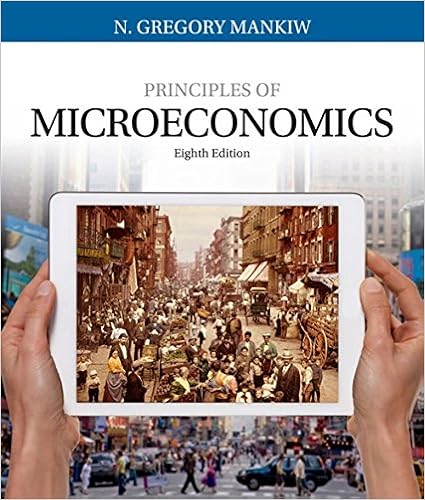# Which of the following is true when there is optimal

• Notes
• 11
• 99% (70) 69 out of 70 people found this document helpful

This preview shows page 5 - 8 out of 11 pages.

##### We have textbook solutions for you!
The document you are viewing contains questions related to this textbook.The document you are viewing contains questions related to this textbook.
Chapter 20 / Exercise 1
Principles of Microeconomics
MankiwExpert Verified
21. Which of the following is true when there is optimal provision of a public good? A) marginal cost = marginal rate of substitution for each consumer B) total costs = total benefits for all consumers C) each consumer's surplus = marginal cost D) total consumer surplus = marginal cost E) the sum of all consumers' marginal rates of substitution = marginal cost
##### We have textbook solutions for you!
The document you are viewing contains questions related to this textbook.The document you are viewing contains questions related to this textbook.
Chapter 20 / Exercise 1
Principles of Microeconomics
MankiwExpert Verified
6 22. If government provision of a public good results in an equal reduction in the privately provided amount of that good, which of the following is true?
23. Which of the following are problems that must be overcome to achieve the Lindahl solution?
24. The property of the independence of irrelevant alternatives refers to which of the following?
25. Which of the following is referred to as the percentage of each medical bill (e.g., 20%) that the patient pays rather than a flat dollar amount? A) out-of-pocket limit B) copayment C) premium D) coinsurance E) deductible
7 PART II: Essay Questions 1. Is moral hazard likely to be a bigger problem in the health insurance market or the life insurance market? Explain.
•••